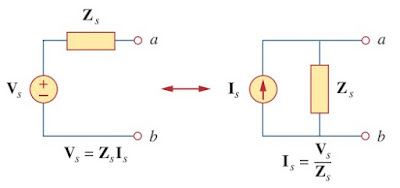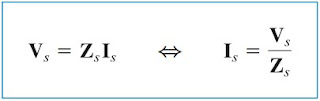omG8HI9LSKFW6lhCj8prTs0Z6lUhdOT9Jhi1Sf4m

# Analyze AC Circuit with Source Transformation

You can see the example of source transformation in the frequency domain involves transforming a voltage source in series with an impedance to a current source in parallel with an impedance, or vice versa.Figure 1. Source transformation
As we go from one source type to another, we must keep following relationship below :(1)

## Source Transformation in AC Circuit

This method helps us to analyze ac circuit with more than one independent sources. The main idea of this method's principle is to substitute voltage source in series with a resistor to a current source in parallel with a resistor or vice versa for dc circuit. Make sure to use impedance instead of resistor when you analyze ac circuit with this method.

This theorem is developed by Jacob Millman. This method simplify multiple parallel sources to an equivalent single resistor. This method is also used for a circuit with an unbalanced bridge circuit.

This method is also known as Millman Theorem. This theorem is useful to simplify a circuit with multi voltage sources or multi current sources to a single source. Even it has unique name, this method also has its basic principle from Ohm's Law and Kirchhoff's Law. But you need to remember, this method only works on a circuit that can be redrawn accordingly. The polarity of all voltage sources in the circuit are referenced to the same point.

Let us cover the technique from the example below :
1. Calculate Vx in the circuit of Figure.(2) using the method of source transformation
We transform the voltage source to a current source and obtain the circuit in Figure.(3a), where
The parallel combination of 5 Ω resistance and (3 + j4) impedance gives
Converting the current source to a voltage source yields the circuit in Figure.(3b),
Related Posts
SHARE